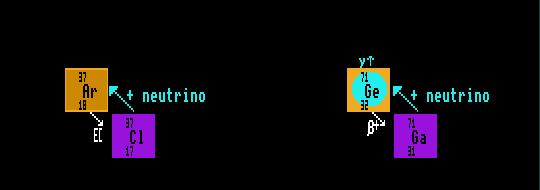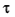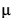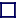### NEUTRINOS

Neutrinos and anti-neutrinos are small, point-like neutral particles emitted in beta decay and inverse beta decay, and interact only in weak-force processes. They have little or no mass, certainly less than 15eV. If massless, they travel at light speed. It is still uncertain whether neutrinos and anti-neutrinos are their own anti-particles. By convention, beta decay is said to involve the emission of anti-neutrinos rather than neutrinos. Only three types of neutrino exist, the electron-, mu- and-neutrinos. Electron anti-neutrinos are emitted in copious amounts by the sun. Because they are both neutral and so tiny most pass through the Earth unhindered, but 1 in 1021 have been detected in huge tanks of perchloroethylene shielded from cosmic rays deep underground: if the energy of the anti-neutrinos is above a certain threshold value (814 keV) they are absorbed by chlorine-37 isotopes in the perchloroethylene which transmutes the chlorine-37 into Argon-37. This process is the reverse of electron capture. For this reaction, the anti-neutrinos have a csa of 10-23 barns. The argon-37 is flushed out with helium gas and concentrated. Its subsequent inverse beta decay with a halflife of 34.8 days back into chlorine-37 releases an auger electron with a distinctive energy spectrum, from the detection of which the original interaction can be inferred.

Similarly, gallium-71 is able to detect lower energy solar electron anti-neutrinos than chlorine, down to 233 keV. The gallium-71 absorbs an anti-neutrino to become germanium-71 in a process the reverse of inverse beta decay which subsequently decays back to gallium-71 with a half life of 11 days.

Neither of these first two neutrino detectors operates in real-time and no directional information can be gleaned from the results; there is no way of knowing where the neutrino originated, or exactly when it came.

 Liquid Scintillation Neutrino Detectors Liquid scintillation neutrino detectors rely on a differing principle. They use a vast fluid-filled under-ground chamber, filled usually with water but not necessarily. Any anti-neutrino coming along might hit a proton within the fluid, and by the reverse of beta decay produces a neutron and a positron. The positron ionizes molecules in the fluid causing it to scintillate, producing a flash of light. The flash is detected by a sensitive photo-multiplier. The newly created neutron, meanwhile, continues onwards until it is captured by another nucleus in a process called neutron capture, causing another flash of light. The near co-incidence in time of these two flashes of light confirms the detection of an anti-neutrino. Such a detector is the Kamikand neutrino detectors in Japan. Unfortunately, although operating in real-time, liquid scintillation neutrino detectors based on water do not give any neutrino directional information because, although it might be thought that the neutrino lies in a straight line joining the source of the two flashes, this is not so because the neutron wanders randomly before being captured by another nucleus. However, by using a liquid containing nuclei with a very high neutron capture cross sectional area, such as gadolinium for instance, this can be done because the gadolinium nuclei grab the neutron almost immediately. (Gadolinium has a very high neutron CSA [48,000 barns], but the isotope xenon-135 beats that by a very long way [2.65 million barns]). The proposal is to use a liquid containing gadolinium tri-chloride, which should be able to pin-point the direction of the absorbed anti-neutrinos. This detector would be suitable to be able to tell if there was a large (10km) nuclear reactor based on plutonium and/or uranium at the centre of the Earth. Although the nuclear fission reactions give off no anti-neutrinos, the decay products will decay by beta decay which do. These will be low-energy anti-neutrinos, of no-where near sufficient energy for the third type of neutrino detector to function. Preliminary measurements of anti-neutrinos emitted from the Earth indicate that there are 16.2 million anti-neutrinos per square centimetre per second streaming out from the Earths core from nuclear reactions, generate about 24TeraWatts of heat (hut it could be as much as 60 TeraWatts, such are the present uncertainties).

 Cerenkov Neutrino Detectors Cerenkov neutrino detectors will only work for very high energy neutrinos, those travelling close to the speed of light. These too are able to deduce the direction from which the neutrino originated. Again, a very large volume of liquid, usually water or sea water, is used as the neutrino interactor, but in this case it is the act of a neutrino travelling through a medium faster than can light that causes the liquid to emit a cone of blue light, called Cerenkov radiation, the result of a kind of electro-magnetic shock wave. Just as with shock waves, the light is emitted as a (hollow) cone, (like a loud-speaker cone), whose apex points to the direction of origin of the neutrino. Since light travels at 0.75c in water, this detector is sensitive to neutrinos travelling at a velocity above 0.75c. Sensitive photo-multipliers are used to detect these flashes of light, which travel out from the apex as an expanding ring of light. Such a detector is the Super-Kamiokand detector in Japan, which consists of an array of 11,000 photo-multiplier tubes scattered over a large area, each one of which may intercept only a small part of any one expanding ring of light. The initial direction of the neutrino is able to be deduced by comparing the timings of such light detections. Un-like the other neutrino detectors, this detector can detect not only electron-neutrinos, but also the other two flavours of neutrino, the-neutrino and the-neutrino.

The sun can generate only electron anti-neutrinos. [See Solar Nucleosynthesis]. Measured at the Earth, the sun emits only a third of the number of the number of electron anti-neutrinos calculated œ. This shortfall can be explained if there are three differing anti-neutrinos (or flavours) each with differing masses, and in traversing space at differing speeds, the identity of the neutrinos continually 'phases in and out' between the three types of neutrino: e-,- and-. If so, their masses would be approximately zero, 1meV and 25eV respectively. This process of continual interchange between flavours of neutrinos is called 'mixing' and would only occur if they all possessed mass, and each mass were different from the other flavour. If all neutrinos had zero mass, then all would travel at the speed of light, and no mixing would be possible. Experimental evidence has now been proved that the three neutrinos do indeed have slight, and differing, masses, and that they do indeed change into one another as they traverse space. The rate of change, or the distance the neutrinos have to traverse in order to change identity, depends upon the difference in their masses; the greater the mass differences the shorter the distances. But different researchers (using alternative methods) have measured great differences in the masses of the three neutrinos, and it could be that the mass of the neutrino depends upon the density of the material through which it is travelling; either through the vacuum of inter-stellar space, or through the Earth. If this turns out to be so, then this could link Dark Energy with neutrinos (although the energy density of Dark Energy has now been measured, the energy density of neutrinos is still un-certain). This would be the only theory to link Dark Energy with particles that are already known rather than hypothetical - except for the fact that the same theoreticians also propose that it is the increase in the acceleron field within higher density materials that gives rise to the gain in mass of the neutrino (and the acceleron is an un-discovered hypothetical particle that bestows the acceleron field!). If they are correct, then as the Universe expands, the mass of individual neutrinos will increase, but as the Universe expands, the number density of neutrinos decreases, leading to an overall decrease in the energy density of the neutrino dark energy field, which will decellerate the expansion of the Universe. This makes enough predictions that can be tested.

By carefully measuring the flux of electron-neutrinos coming from the Sun with one liquid-scintillation detector, and the sum of all three types of neutrino with another type of liquid scintillation detector, the Sudbury Neutrino Observatory have confirmed beyond any doubt that only one-third of the electrons detected from the Sun are electron-neutrinos, the rest being either mu-neutrinos or tau-neutrinos. This proves that the Sun is powered by thermo-nuclear fusion.

[œ Only electron anti-neutrinos can be detected with our present experiments; we would need strange/charm baryonic matter to detect the mu-anti-neutrinos, and top/bottom baryonic matter to detect the tau-anti-neutrinos, tons of each, but we have none!: Only ordinary up/down baryonic matter is stable, and only then when the un-stable neutrons are gathered next to protons in atomic nuclei].

Neutrinos out-number photons in the Universe by 1000 Million to one, the same factor that photons out-number baryons by. Therefore, if neutrinos have a mass, however slight, then they could contribute significantly to the mass of the Universe.

Unlike all other particles, Neutrinos are thought to exist only in left-handed form [and anti-neutrinos only in right-handed form]. See 'Higgs and Left-handed [and right-handed? neutrinos'].

The masses of the 3 neutrinos are:
Electon Neutrino: νe < 1.1 eV
Muon Neutrino: νμ < 1.7 MeV
Tau Neutrino: ντ < 15.5 MeV